# Kraken Daily Market Report for January 26 2021

## Overview

• Total spot trading volume at $1.33 billion, near the 30-day average of$1.65 billion.
• Total futures notional at $542.6 million. • The top 5 traded coins were, respectively, Bitcoin, Ethereum, Tether, Polkadot, and Chainlink. • Although Chainlink (-1.0%), Litecoin (-1.7%) and others were down; Bitcoin(+1.2%), Ethereum (+3.3%), and Uniswap (+23%) were up. January 26, 2021$1326.2M traded across all markets today
Crypto, EUR, USD, JPY, CAD, GBP, CHF, AUD
XBT
$32529. ↑1.2%$552.5M
ETH
$1358.7 ↑3.3%$442.4M
USDT
$1.0011 ↑0.1%$149.3M
DOT
$17.117 ↓0.4%$40.4M
$23.059 ↓1.0%$25.0M
USDC
$0.9999 ↑0.0%$20.2M
BCH
$427.28 ↓0.9%$19.6M
UNI
$13.853 ↑23%$18.5M
$0.3441 ↑0.7%$16.7M
LTC
$134.74 ↓1.7%$14.6M
XRP
$0.2681 ↑0.3%$13.2M
AAVE
$277.08 ↑13%$8.43M
XLM
$0.2614 ↑0.3%$5.41M
XTZ
$2.9084 ↓0.26%$4.2M
FIL
$22.180 ↓1.0%$3.61M
CRV
$2.1399 ↑7.2%$3.43M
ALGO
$0.6021 ↑8.6%$3.36M
SNX
$16.771 ↑8.6%$3.2M
XMR
$137.92 ↑0.6%$2.92M
DAI
$1.0005 ↑0.02%$2.64M
GRT
$0.5361 ↑1.5%$2.22M
EOS
$2.6297 ↓0.6%$2.18M
ICX
$0.8948 ↑11%$2.08M
COMP
$236.97 ↑12%$1.95M
ATOM
$7.7332 ↑0.29%$1.82M
YFI
$30280. ↑3.6%$1.69M
KEEP
$0.3131 ↓4.2%$1.5M
KSM
$102.03 ↑3.4%$1.33M
BAT
$0.2989 ↓3.0%$1.28M
OMG
$3.4422 ↓2.7%$1.19M
DASH
$104.90 ↑0.4%$1.19M
TRX
$0.0295 ↑0.2%$1.11M
XDG
$0.0082 ↓0.7%$1.09M
QTUM
$3.4386 ↑4.6%$1.04M
MLN
$36.117 ↓5.6%$1.01M
NANO
$3.2120 ↑1.2%$1.0M
MANA
$0.1665 ↑1.0%$916K
ZEC
$89.189 ↑1.6%$914K
WAVES
$6.8173 ↑2.5%$905K
KAVA
$2.3313 ↓1.5%$643K
ETC
$7.4335 ↓0.7%$520K
STORJ
$0.4256 ↑9.9%$393K
KNC
$1.3156 ↓0.04%$355K
OXT
$0.2995 ↑1.7%$288K
ANT
$4.0750 ↑5.1%$270K
SC
$0.0044 ↓1.2%$266K
PAXG
$1869.4 ↑0.3%$246K
REP
$21.454 ↑4.4%$221K
LSK
$1.3000 ↓1.6%$187K
GNO
$115.59 ↑0.8%$177K
BAL
$21.730 ↑3.7%$127K
REPV2
$19.177 ↓0.6%$59.7K
TBTC
$33172. ↓2.6%$56.2K

#####################. Trading Volume by Asset. ##########################################

The figures below break down the trading volume of the largest, mid-size, and smallest assets. Cryptos are in purple, fiats are in blue. For each asset, the chart contains the daily trading volume in USD, and the percentage of the total trading volume. The percentages for fiats and cryptos are treated separately, so that they both add up to 100%.

Figure 1: Largest trading assets: trading volume (measured in USD) and its percentage of the total trading volume (January 26 2021)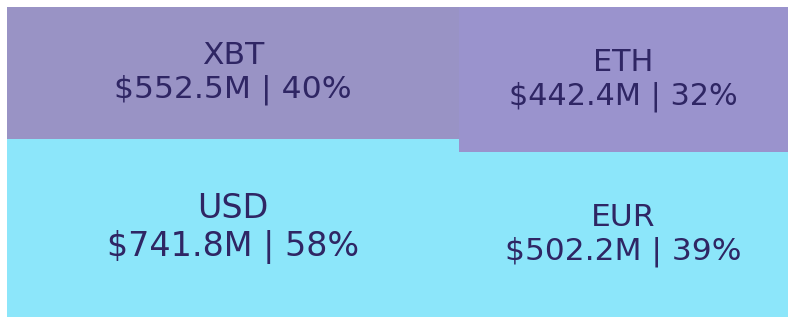Figure 2: Mid-size trading assets: (measured in USD) (January 26 2021)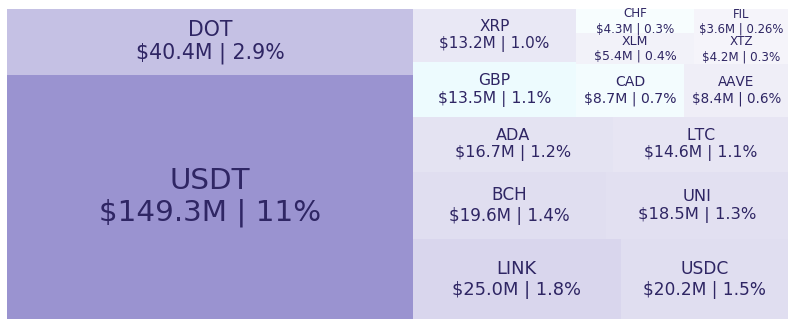Figure 3: Smallest trading assets: (measured in USD) (January 26 2021)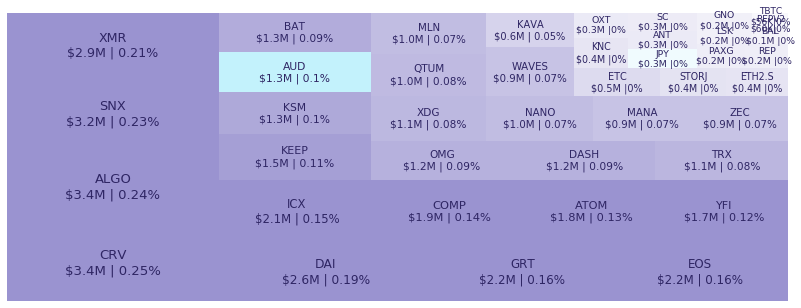Spread percentage is the width of the bid/ask spread divided by the bid/ask midpoint. The values are generated by taking the median spread percentage over each minute, then the average of the medians over the day.

Figure 4: Average spread % by pair (January 26 2021)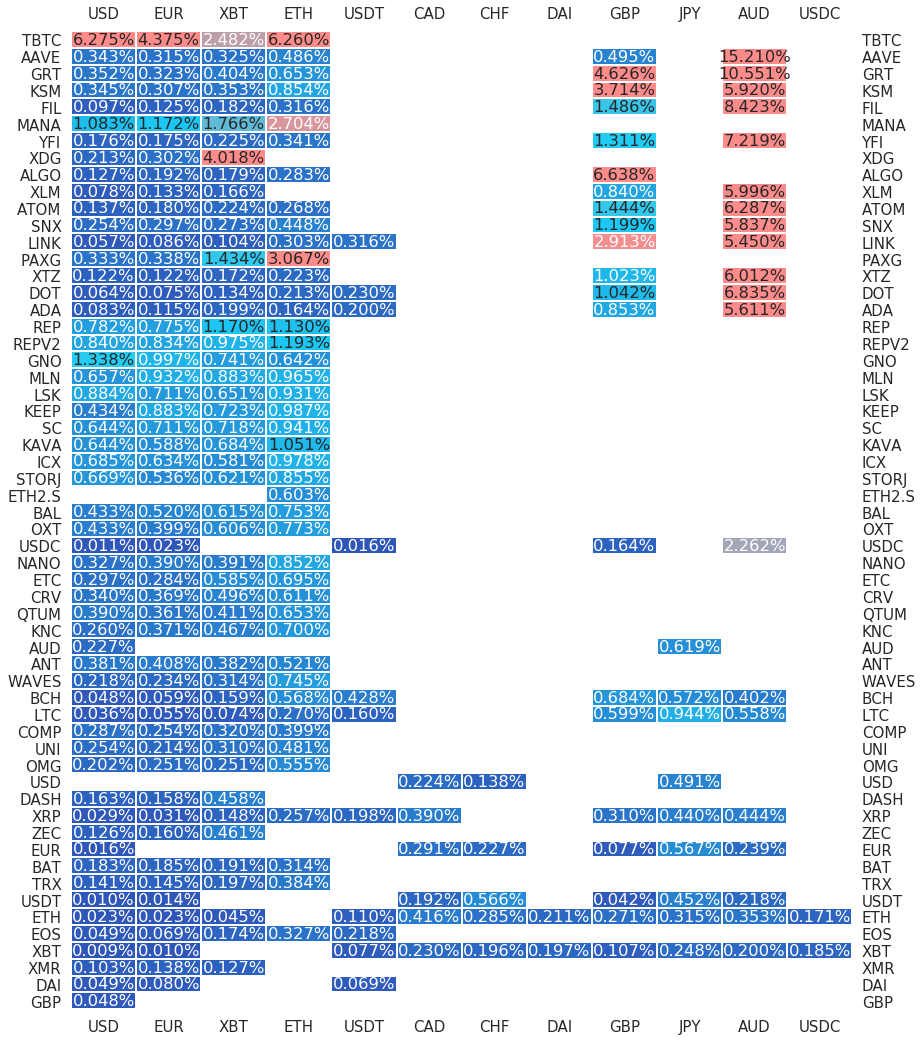.

#########. Returns and Volume ############################################

## Returns and Volume

Figure 5: Returns of the four highest volume pairs (January 26 2021)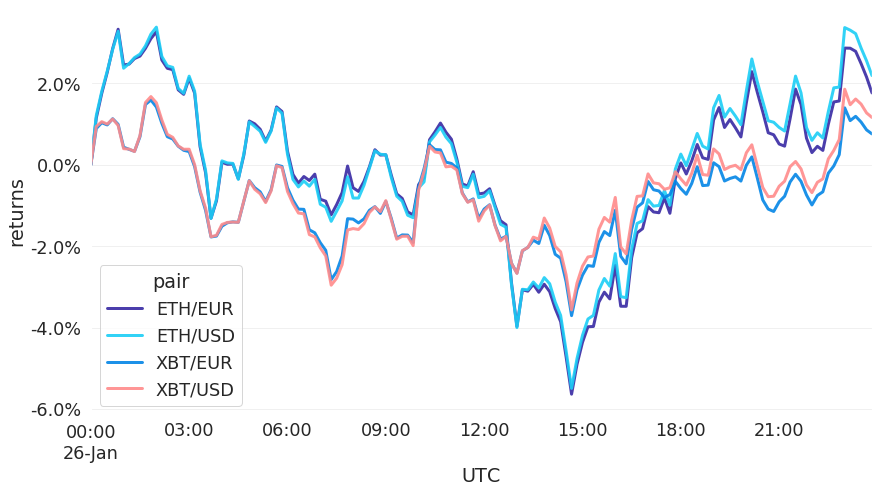Figure 6: Volume of the major currencies and an average line that fits the data to a sinusoidal curve to show the daily volume highs and lows (January 26 2021)